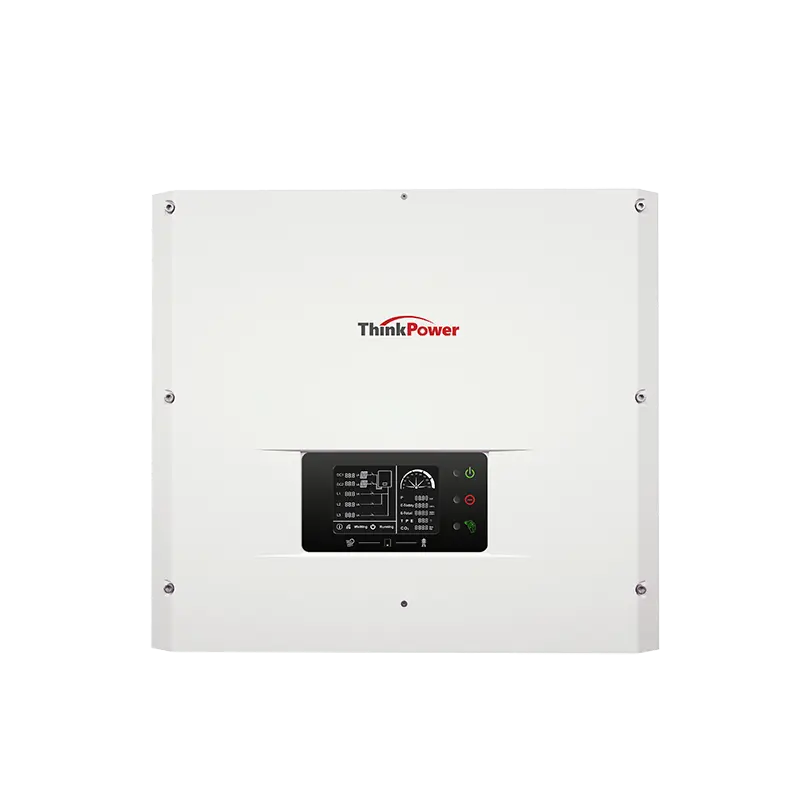## The difference between single-phase inverter and three phase inverter

Difference between single-phase inverter and three-phase inverter

1. Single-phase inverter

A single-phase inverter converts a DC input into a single-phase output. The output voltage/current of a single-phase inverter is only one phase, and its nominal frequency is 50HZ or 60Hz nominal voltage. The nominal voltage is defined as the voltage level at which an electrical system operates. There are different nominal voltages, i.e. 120V, 220V, 440V, 690V, 3.3KV, 6.6KV, 11kV, 33kV, 66kV, 132kV, 220kV, 400kV, and 765kV(Related post on these numbers: Why power transmission is a multiple of 11, That is 11kV, 22kV, 66kV, etc.?

Low nominal voltages can be achieved directly through the inverter using an internal transformer or step-up booster circuit, while for high nominal voltages an external booster transformer is used.

Single-phase inverters are used for low loads. Compared with the three-phase inverter, the single-phase loss is larger and the efficiency is lower. Therefore, three-phase inverters are preferred for high loads.

2. Three-phase inverter

Three-phase inverters convert DC into three-phase power. The three-phase power supply provides three alternating current with evenly separated phase angles. All three waves generated at the output end have the same amplitude and frequency, but are slightly different due to the load, while each wave has a 120o phase shift between each other.

Basically, a single three-phase inverter is 3 single-phase inverters, where each inverter is 120 degrees out of phase, and each single-phase inverter is connected to one of three load terminals.

Content Browse: What is the three-phase inverter, what is the role

There are different topologies for constructing three-phase voltage inverter circuits. If it is a bridge inverter, running the switch in 120 degree mode the operation of the three-phase inverter makes each switch operate for a total time of T/6, which produces an output waveform with 6 steps. There is a zero voltage step between the positive and negative voltage levels of the square wave.

The inverter power rating can be further increased. In order to build an inverter with a high power rating, 2 inverters (three-phase inverters) are connected in series to obtain a high voltage rating. For high current ratings, 2 6-step 3 inverters can be connected.

### Single Phase S1000TL-S6000TL### Grid Tie Inverter TP10KTL-TP15KTL

Post time: Sep-07-2023